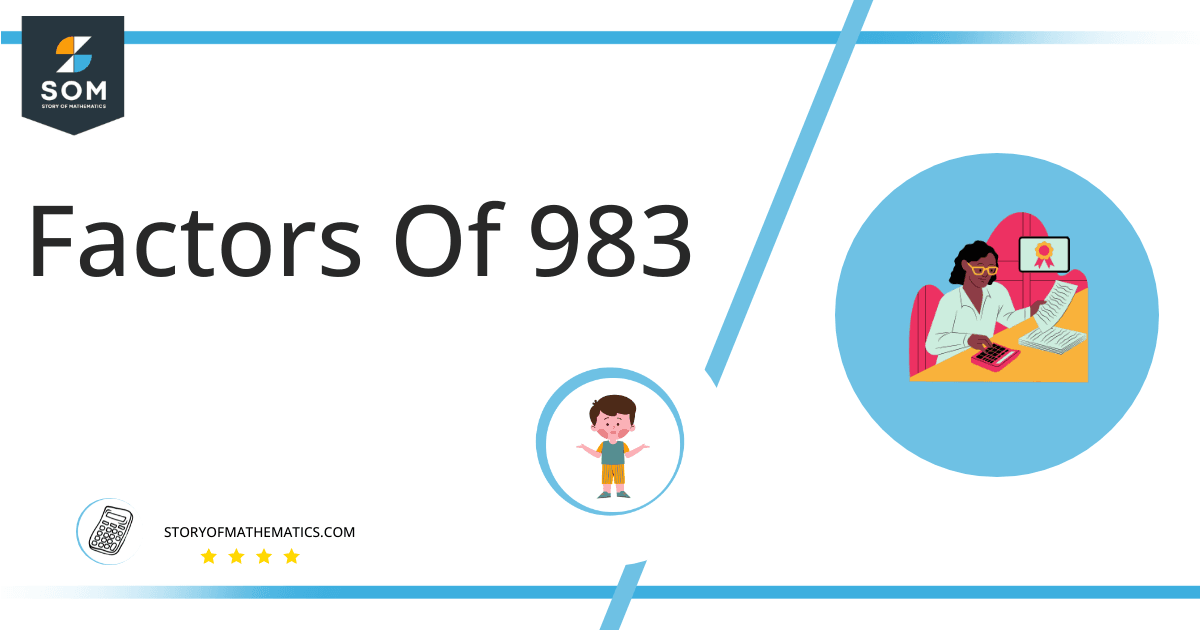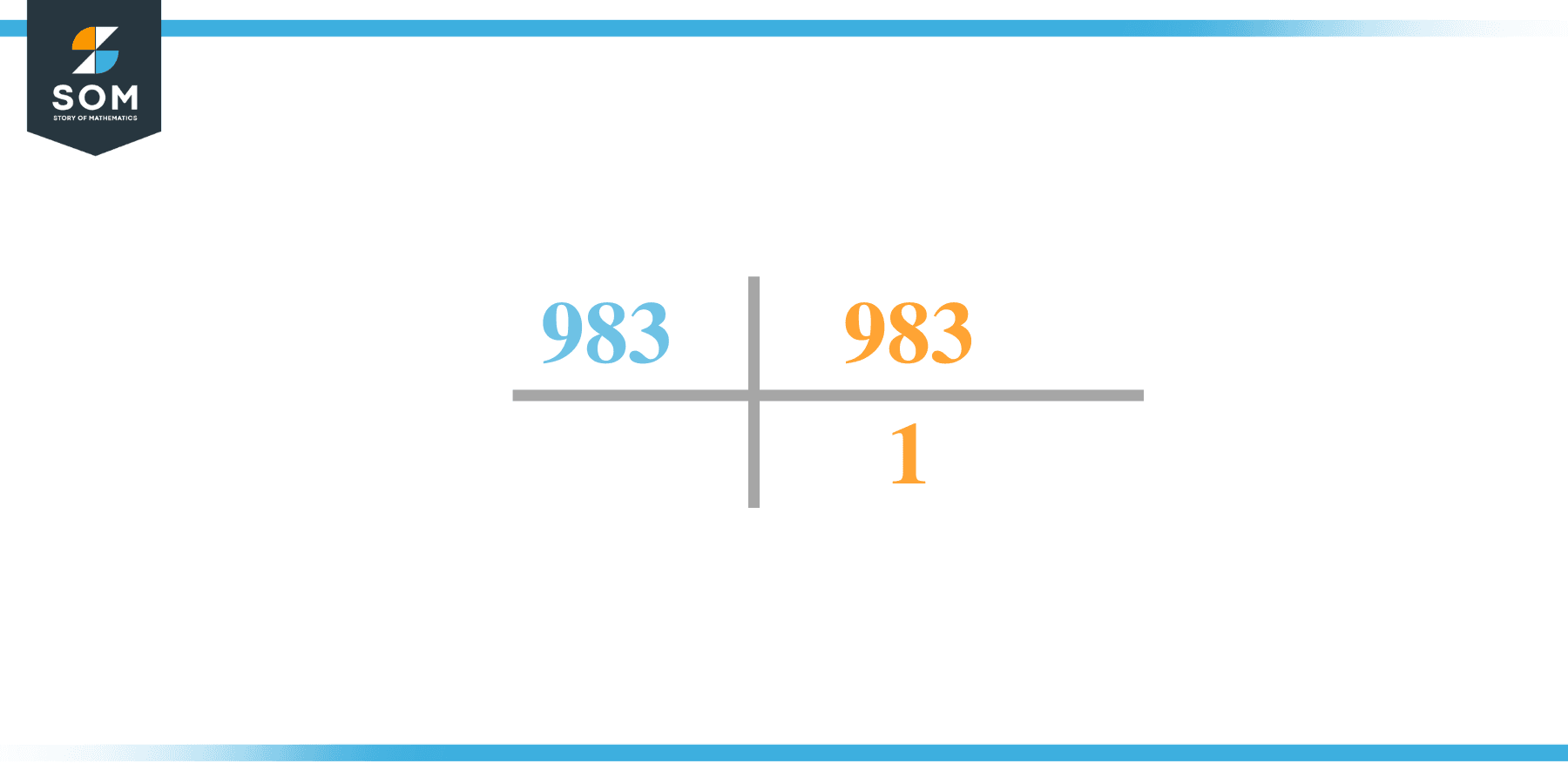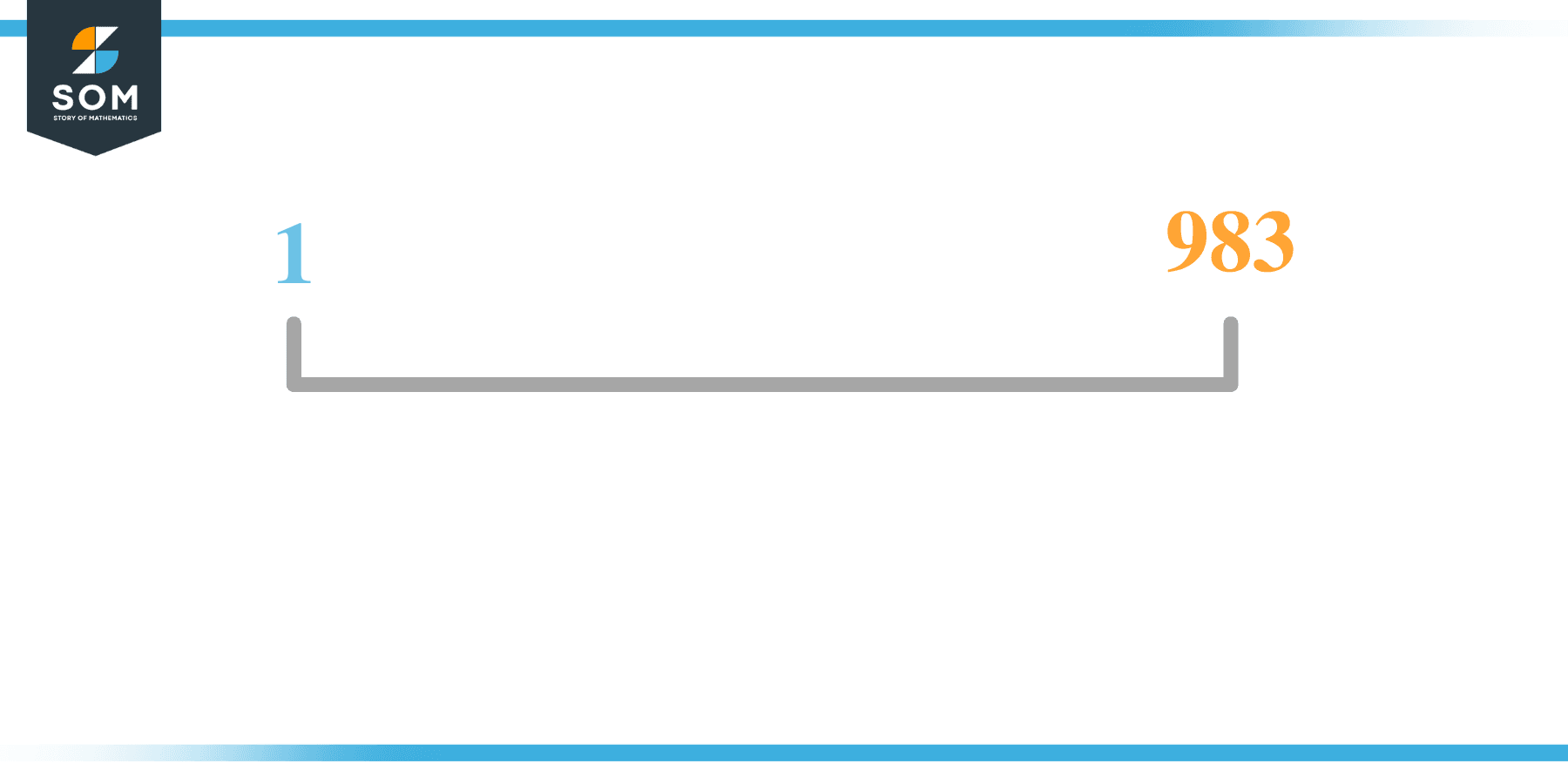# Factors of 983: Prime Factorization, Methods, and Examples

The factors of 983 are the numbers that give off zero as the remainder when 983 is the dividend for such numbers.The given number’s factors can be positive and negative, provided that the given number is achieved upon multiplication of two-factor integers.

### Factors of 983

Here are the factors of number 983.

Factors of 983: 1 and 983

### Negative Factors of 983

The negative factors of 983 are similar to its positive aspects, just with a negative sign.

Negative Factors of 983: -1 and -983

### Prime Factorization of 983

The prime factorization of 983 is the way of expressing its prime factors in the product form.

Prime Factorization: 1 x 983

In this article, we will learn about the factors of 983 and how to find them using various techniques such as upside-down division, prime factorization, and factor tree.

## What Are the Factors of 983?

The factors of 983 are 1 and 983. These numbers are the factors as they do not leave any remainder when divided by 983.

The factors of 983 are classified as prime numbers. The prime factors of the number 983 can be determined using the prime factorization technique.

## How To Find the Factors of 983?

You can find the factors of 983 by using the rules of divisibility. The divisibility rule states that any number, when divided by any other natural number, is said to be divisible by the number if the quotient is the whole number and the resulting remainder is zero.

To find the factors of 983, create a list containing the numbers that are exactly divisible by 983 with zero remainders. One important thing to note is that 1 and 983 are the 983’s factors as every natural number has 1 and the number itself as its factor.

1 is also called the universal factor of every number. The factors of 983 are determined as follows:

$\dfrac{983}{1} = 983$

$\dfrac{983}{983} = 1$

Therefore, 1 and 983 are the factors of 983.

### Total Number of Factors of 983

For 983, there are 2 positive factors and 2 negative ones. So in total, there are 4 factors of 983.

To find the total number of factors of the given number, follow the procedure mentioned below:

1. Find the factorization of the given number.
2. Demonstrate the prime factorization of the number in the form of exponent form.
3. Add 1 to each of the exponents of the prime factor.
4. Now, multiply the resulting exponents together. This obtained product is equivalent to the total number of factors of the given number.

By following this procedure, the total number of factors of 983 is given as:

Factorization of 983 is 1 x 983.

The exponent of all factors is 1.

Adding 1 to each and multiplying them together results in 4.

Therefore, the total number of factors of 983 is 4. 2 are positive, and 2 factors are negative.

### Important Notes

Here are some essential points that must be considered while finding the factors of any given number:

• The factor of any given number must be a whole number.
• The factors of the number cannot be in the form of decimals or fractions.
• Factors can be positive as well as negative.
• Negative factors are the additive inverse of the positive factors of a given number.
• The factor of a number cannot be greater than that number.
• Every even number has 2 as its prime factor, the smallest prime factor.

## Factors of 983 by Prime FactorizationThe number 983 is a prime number. Prime factorization is a valuable technique for finding the number’s prime factors and expressing the number as the product of its prime factors.

Before finding the factors of 983 using prime factorization, let us find out what prime factors are. Prime factors are the factors of any given number that are only divisible by 1 and themselves.

To start the prime factorization of 983, start dividing by its most minor prime factor. First, determine that the given number is either even or odd. If it is an even number, then 2 will be the smallest prime factor.

Continue splitting the quotient obtained until 1 is received as the quotient. The prime factorization of 983 can be expressed as:

983 = 1 x 983

## Factors of 983 in PairsThe factor pairs are the duplet of numbers that, when multiplied together, result in the factorized number. Factor pairs can be more than one depending on the total number of factors given.

For 983, the factor pairs can be found as:

1 x 983 = 983

The possible factor pair of 983 is given as (1, 983).

All these numbers in pairs, when multiplied, give 983 as the product.

The negative factor pair of 983 is given as:

-1 x -983 = 983

It is important to note that in negative factor pairs, the minus sign has been multiplied by the minus sign, due to which the resulting product is the original positive number. Therefore, -1 and -983 are called negative factors of 983.

The list of all the factors of 983, including positive as well as negative numbers, is given below.

Factor list of 983: 1, -1, 983, and -983

## Factors of 983 Solved Examples

To better understand the concept of factors, let’s solve some examples.

### Example 1

How many factors of 983 are there?

### Solution

The total number of Factors of 983 is 2.

Factors of 983 are 1 and 983.

### Example 2

Find the factors of 983 using prime factorization.

### Solution

The prime factorization of 983 is given as:

983 $\div$ 983 = 1

So the prime factorization of 983 can be written as:

1 x 983 = 983